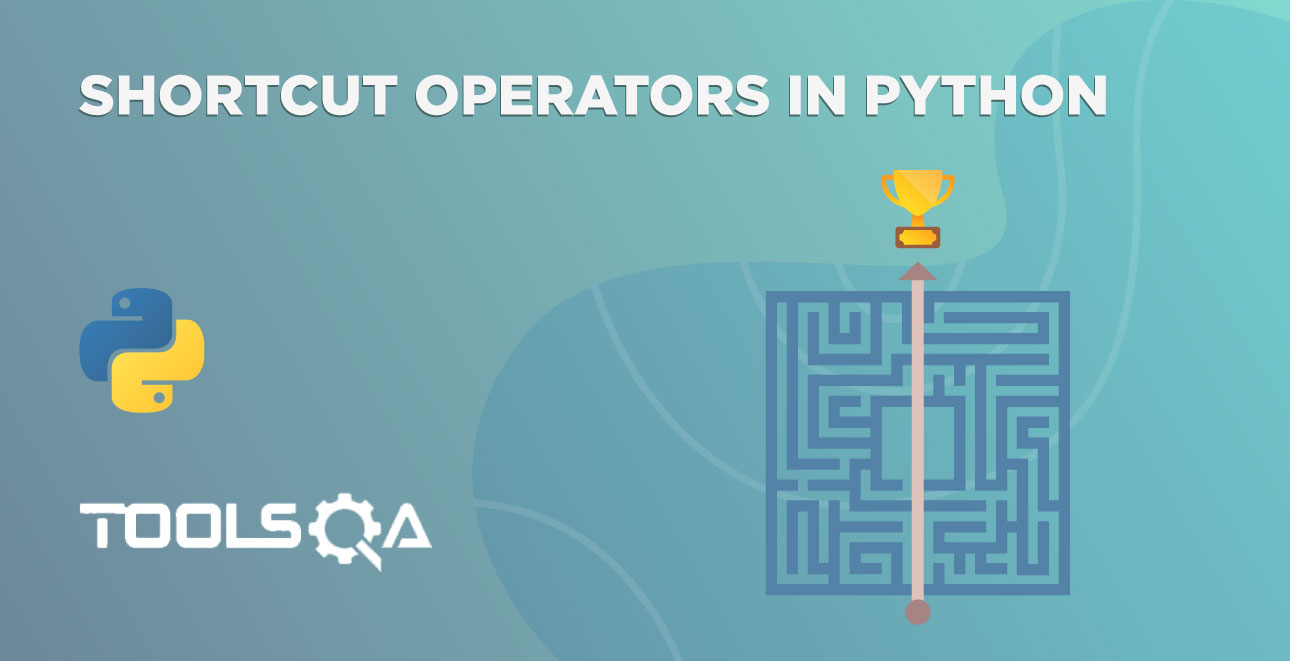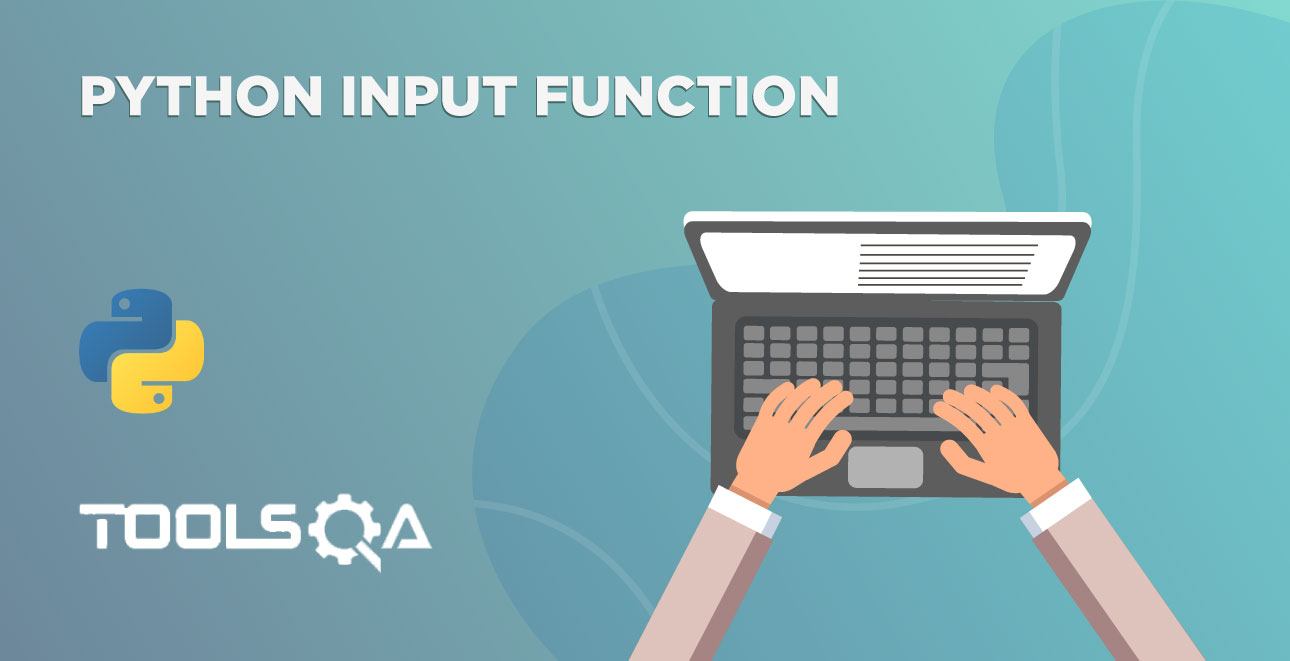We have learned about the Python shortcut operators in the previous tutorial that reduces the length of the expression and increases the readability. In this tutorial, we will discuss how to make our code readable and easy to understand for others by using Comments in Python. You are going to learn the following topics in this tutorial.

• What are Comments in Code?
• Why are comments beneficial during coding?
• How to write Single line Comments in Python?
• How to write Multiple line Comments in Python?

## What are Comments in Code?

Every programming language has a concept called comments. What are these comments? And what's the use of these comments in the code?

Comments are the statements that are ignored by the interpreter or compiler at runtime.  If you have comments in the code, then the compiler ignores those statements. Moreover, every coding language has its syntax, which differentiates a comment from actual code.

### Why are comments beneficial during coding?

• Future Reference - Assume that you have written a program which contains several hundred lines of code without any comments. If you open the program after one or two months, then you will find it challenging to understand your code. Additionally, in most cases, we will forget the role of a code block, and again we have to trace it to understand. So putting a comment in code saves a lot of time when you have to update the code again.

• Comments help to understand the code of others - We usually work in a team structure, where others review the code of one team member. Also, you might need to work on a feature that was earlier worked by some other developer. In both cases, you will need to understand the code that someone else has written. If you have used comments in your code, it makes the life of the other person looking at your code much easier. He can do a useful review, and also chances of defects are much lesser if someone else is editing your code.

• Code Debugging - Sometimes, we have to check a part of the code to see whether it's working as expected or not. In this case, we can comment on the remaining code. Without comments, we will have to remove the code to check the output. If it's not working, then we have to enter the same code again. It's a pretty time-consuming process.

Now we know the comments, and it's importance, let's take a look at the syntax of the comments in Python.

## How to write comments in Python?

In Python, any statement which begins with a hash (#) symbol is a comment. So, any code you write after the hash (#) symbol doesn't get executed.

We can write comments at any point in the program. See the examples.

``````# variable to store the name of the company
toolsqa = 'ToolsQA'
``````

The first line in the code is a comment that explains the variable below. You won't get any output if you run the above code as we didn't print anything. Let's write a program to add two numbers with comments.

``````# initializing two variables
a = 5
b = 7

result = a + b

# printing the result of the addition
print(result)
``````

Even non-programmers able to understand the above code. The interpreter ignores all the statements that begin with a hash (#) symbol. Open the PyCharm and run the above program.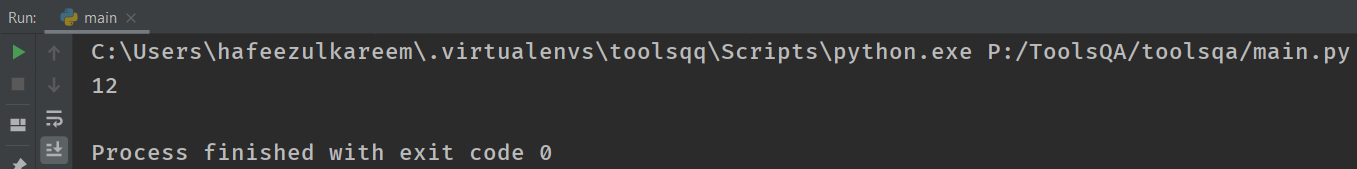Commenting the code is good practice. Make it as a habit. We are going to practice more in the following tutorials. Maybe you feel commenting in the above program is an overdose. But for beginners, it's a good practice to comment even for the small statements. Once you are familiar with the programming, then use comments for a specific block of code at once. Let's see an example of code debugging.

``````# initializing variables
a = 5
b = 7

# result = a + b
d = b - a

# printing the value
print(d)
``````

We made the result = a + b as a comment in the program. Here, we are checking the other piece of code. We may see the result in the next steps. So, we didn't remove it.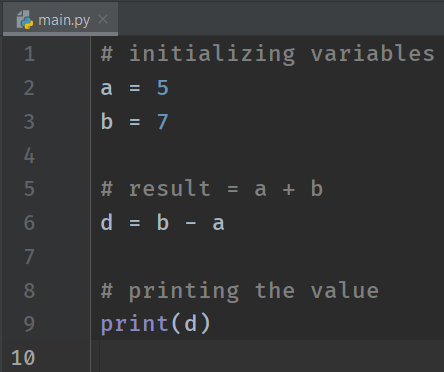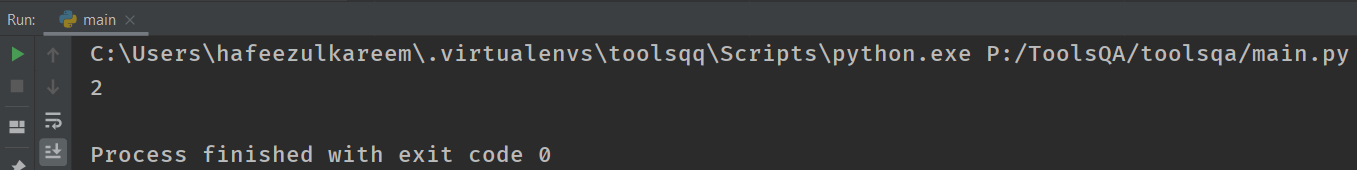### Multiple Line Comments in Python

What if you want to write a comment on multiple lines? Is it possible in Python?

Yeah!  We can put a hash (#) symbol in front of each line for multi-line commenting. Let's see an example.

``````# Read the input from the user
# Perform some operations on the input data
# Output the result
print("Multi-line commenting")
``````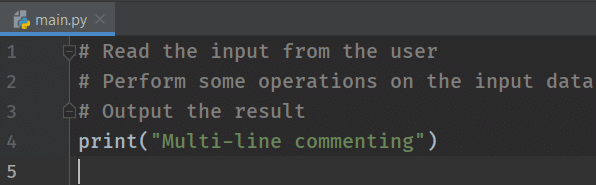Conclusively, that's it for the tutorial. Let's wrap it up here.

## Key Takeaways

1. Firstly, the Python interpreter will ignore the comments at the runtime of the program.
2. Additionally, we will use hash (#) sign to begin a comment in Python.
3. Moreover, use hash (#) in front of each line for multi-line comments.
4. Comments help to recognize the functionality of a code block.
5. Additionally, it's useful when debugging the code.
6. Lastly, comments act as a reference for the future.

## Practice Yourself

``````
#Program 1

print("#Hello", end=' ')
#print("ToolsQA")
print("#Python")
``````
``````#Program 2

# This program
print(2 + 5)
``````
``````#Program 3

# a = 5
# a += 1
print(a)
``````
``````#Program 4

num = 5
# num = 0
print(num)
``````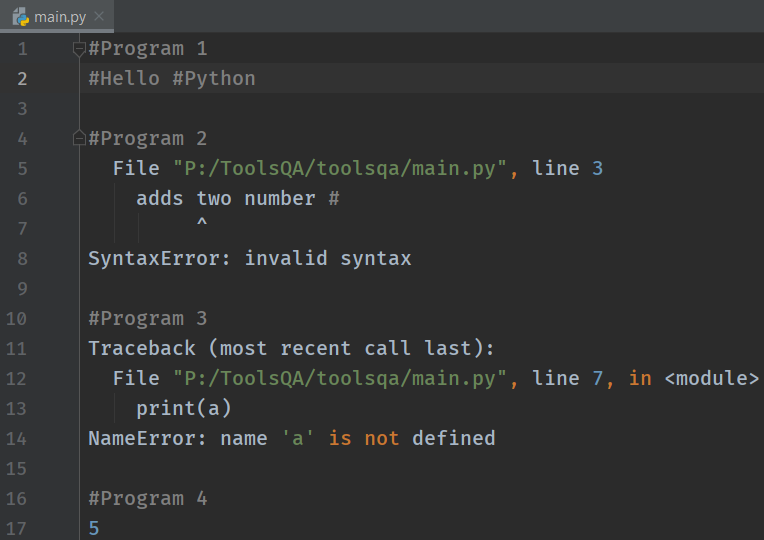## What Next?

To conclude, we have learned how to comment on the code in Python. It's one of the essential parts of coding. So, make sure that you comment on your code. Additionally, it saves a lot of time in reference.

Till now, we have hardcoded the values to the variables and expressions. In the next tutorial, we will see how to take data from the user using the Python Input Function.

Happy Coding :)